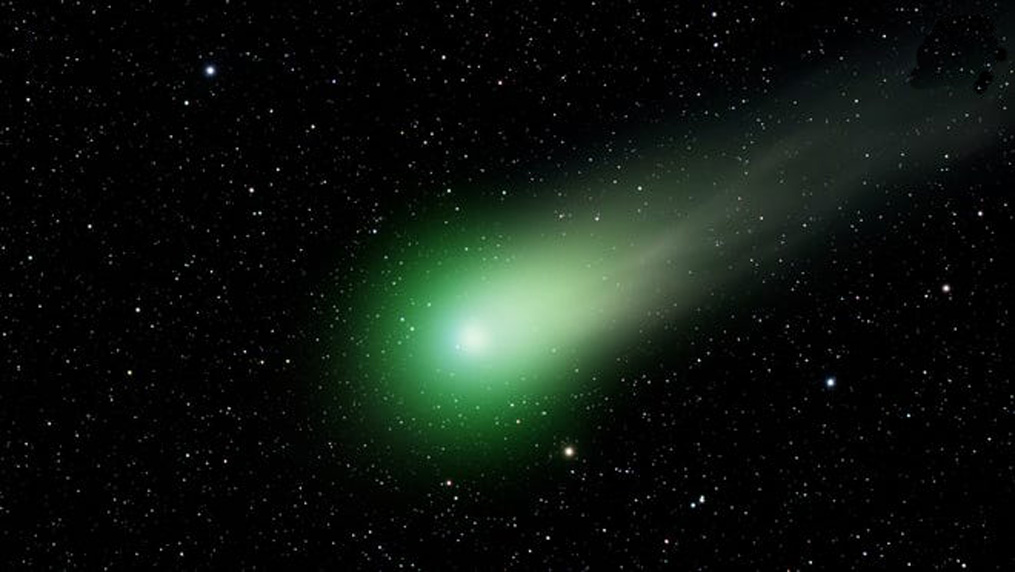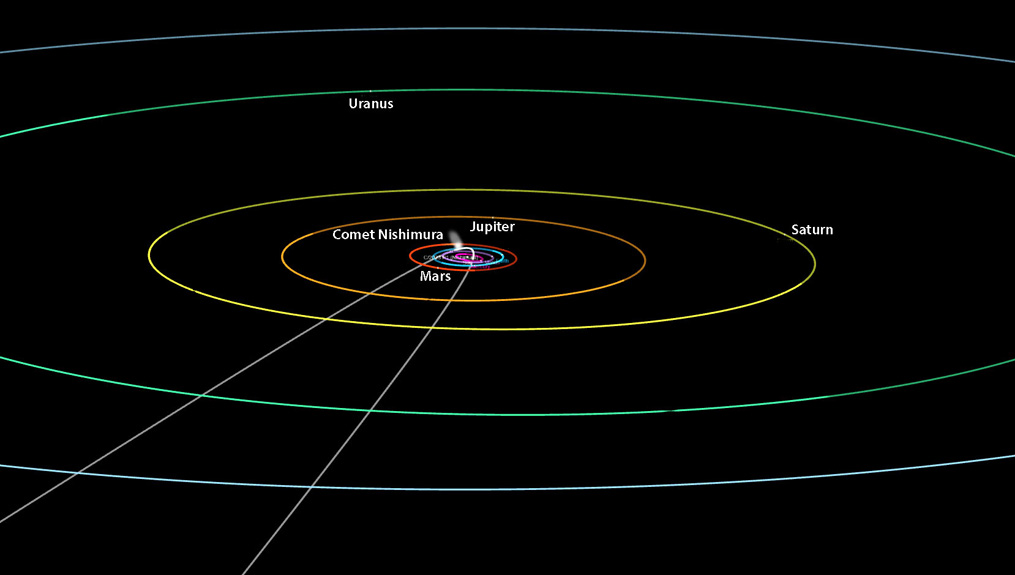Comet Nishimura ephemeris 1600-2500 AD Comet Nishimura (C/2023 P1) named after its discoverer Hideo Nishimura was discovered on August 12, 2023 and will come closest to us in mid-September of 2023. It's last perihelion was in July of 1588 AD. It has an interesting orbit of some 434 years and a high inclination to the ecliptic of 132 degrees. At perihelion it comes just inside the orbit of Mercury.It looks a lot (greenish) like comet Ison back in 2013, but has a much shorter orbit, and like all comets portends that some significant event is about to occur on the world scene. It spends most of its time in tropical Leo or Cancer and travels retrograde through the signs except during close approaches. Most of us alive today have it in the sign of tropical Cancer.Below is a long-term ephemeris of the comet between 1600 and 2500 AD (derived from the Jet Propulsion Horizons website). Nishimura(C/2023 P1) ephemeris Midnight UT 1600 Jan 01 = 09Leo45 1660's to 1680's Leo/Cancer 1700 Jan 01 = 29Can19 1800 Jan 01 = 28Can07 1900 Jan 01 = 27Can11 1910 Jan 01 = 27Can02 1920 Jan 01 = 26Can54 1930 Jan 01 = 26Can42 1940 Jan 01 = 26Can29 1950 Jan 01 = 26Can14 1960 Jan 01 = 25Can56 1970 Jan 01 = 25Can33 1980 Jan 01 = 25Can04 1990 Jan 01 = 24Can22 2000 Jan 01 = 23Can22 2010 Jan 01 = 21Can34 2020 Jan 01 = 15Can55 2022 Jan 01 = 10Can57 2023 Jan 01 = 02Can32 2023 Jan 07 = 30Gem00 2023 Feb 01 = 21Gem07 2023 Mar 01 = 14Gem25 2023 Apr 01 = 13Gem01 2023 May 01 = 15Gem45 2023 Jun 01 = 21Gem20 2023 Jul 01 = 28Gem51 2023 Jul 05 = 00Can00 2023 Aug 01 = 09Can50 2023 Aug 28 = 00Leo00 2023 Sep 01 = 06Leo47 2023 Sep 09 = 00Vir00 2023 Sep 15 = 22Vir49 2023 Sep 16 = 00Lib00 2023 Oct 01 = 18Lib59 2023 Nov 01 = 25Lib50 2023 Dec 01 = 29Lib48 2023 Dec 04 = 00Sco00 2023 Dec 26 = 30Lib00 2024 Jan 01 = 29Lib18 2024 Feb 01 = 16Lib30 2024 Feb 18 = 30Vir00 2024 Mar 01 = 16Vir50 2024 Mar 19 = 30Leo00 2024 Apr 01 = 22Leo27 2024 May 01 = 16Leo18 2024 Jun 01 = 17Leo57 2024 Jul 01 = 22Leo34 2024 Aug 01 = 28Leo26 2024 Aug 9/10 = 00Vir00 2024 Sep 01 = 04Vir26 2024 Oct 01 = 09Vir29 2024 Nov 01 = 13Vir10 2024 Dec 01 = 14Vir03 2025 Jan 01 = 10Vir51 2026 Jan 01 = 00Vir26 2026 Jan 04 = 30Leo00 2027 Jan 01 = 25Leo33 2028 Jan 01 = 22Leo34 2029 Jan 01 = 20Leo26 2030 Jan 01 = 18Leo54 2035 Jan 01 = 14Leo33 2040 Jan 01 = 12Leo25 2050 Jan 01 = 10Leo03 2100 Jan 01 = 06Leo18 2200 Jan 01 = 04Leo22 2300 Jan 01 = 03Leo15 2376 to 2410 = Leo/Can 2400 Jan 01 = 00Leo01 2500 Jan 01 = 12Leo21

back to table
back to home